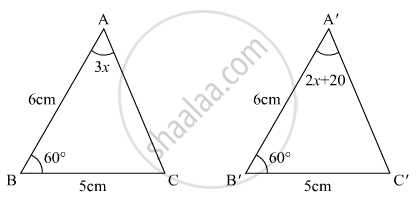# In the Given Figure, the Measure of ∠B'A'C' is - Mathematics

MCQ

In the given figure, the measure of ∠B'A'C' is•  50°

•  60°

•  70°

•  80°

#### Solution

We have to find  B’A’C’ince triangles are congruent

So ∠A = ∠A

3X = 2X + 20

X = 20

Now in  A’B’C’

∠A + ∠B + C  = 180° (By property of triangle)

60° + 60° + ∠C = 180°

∠C = 60°

∠A = 2X + 20

= 2 xx 20° + 20°

= 40° + 20

= 60°

Hence (b) B’A’C’ = 60°.

Concept: Congruence of Triangles
Is there an error in this question or solution?

#### APPEARS IN

RD Sharma Mathematics for Class 9
Chapter 12 Congruent Triangles
Exercise 12.8 | Q 11 | Page 86
Share